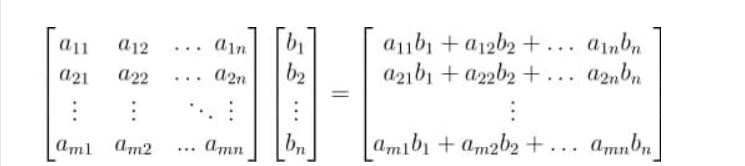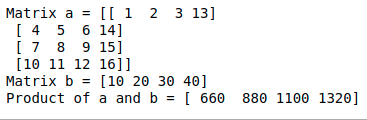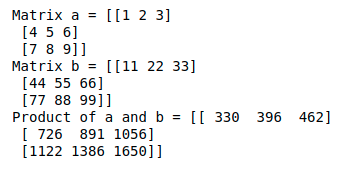# Parallel matrix-vector multiplication in NumPy

• Last Updated : 23 Sep, 2021

In this article, we will discuss how to do matrix-vector multiplication in NumPy.

## Matrix multiplication with Vector

For a matrix-vector multiplication, there are certain important points:

Attention geek! Strengthen your foundations with the Python Programming Foundation Course and learn the basics.

To begin with, your interview preparations Enhance your Data Structures concepts with the Python DS Course. And to begin with your Machine Learning Journey, join the Machine Learning - Basic Level Course

• The end product of a matrix-vector multiplication is a vector.
• Each element of this vector is obtained by performing a dot product between each row of the matrix and the vector being multiplied.
• The number of columns in the matrix is equal to the number of elements in the vector.```# a and b are matrices
prod = numpy.matmul(a,b)```

For matrix-vector multiplication, we will use np.matmul() function of NumPy, we will define a 4 x 4 matrix and a vector of length 4.

## Python3

 `import` `numpy as np`` ` `a ``=` `np.array([[``1``, ``2``, ``3``, ``13``],``              ``[``4``, ``5``, ``6``, ``14``],``              ``[``7``, ``8``, ``9``, ``15``],``              ``[``10``, ``11``, ``12``, ``16``]])`` ` `b ``=` `np.array([``10``, ``20``, ``30``, ``40``])`` ` `print``(``"Matrix a ="``, a)``print``(``"Matrix b ="``, b)``print``(``"Product of a and b ="``,``      ``np.matmul(a, b))`

Output:## Matrix multiplication with another Matrix

We use the dot product to do matrix-matrix multiplication. We will use the same function for this also.

`prod = numpy.matmul(a,b)  # a and b are matrices`

For a matrix-matrix multiplication, there are certain important points:

• The number of columns in the first matrix should be equal to the number of rows in the second matrix.
• If we are multiplying a matrix of dimensions m x n with another matrix of dimensions n x p, then the resultant product will be a matrix of dimensions m x p

We will define two 3 x 3 matrix:

## Python3

 `import` `numpy as np`` ` `a ``=` `np.array([[``1``, ``2``, ``3``],``              ``[``4``, ``5``, ``6``],``              ``[``7``, ``8``, ``9``]])`` ` `b ``=` `np.array([[``11``, ``22``, ``33``],``              ``[``44``, ``55``, ``66``],``              ``[``77``, ``88``, ``99``]])`` ` `print``(``"Matrix a ="``, a)``print``(``"Matrix b ="``, b)``print``(``"Product of a and b ="``, np.matmul(a, b))`

Output:My Personal Notes arrow_drop_up